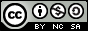- 目录 -
LeetCode 11 盛最多水的容器(双指针)

**说明：**你不能倾斜容器，且 n 的值至少为 2。输入：[1,8,6,2,5,4,8,3,7]



## 代码

  1 2 3 4 5 6 7 8 9 10 11 12 13 14 15 16 17 18 19  class Solution { public: int maxArea(vector &height) { // s = (r-l)*min(a[l],a[r]) ,求 max(s) int maxx = 0, l = 0, r = height.size() - 1; while (r - l >= 1) { int s = (r - l) * min(height[l], height[r]); maxx = max(maxx, s); if (height[l] > height[r]) r--; else l++; } return maxx; } };